Copy the CurrentRegion of a cell of each sheet into one sheet using VBA in Microsoft Excel

If you are handling multiple sheets at one time, and you want to copy data from each sheet into a master worksheet then you should read this article. We will use currentregion property of VBA code to consolidate data from all the worksheets into a single sheet. This property is useful for many operations that automatically expand the selection to include the entire current region, such as the AutoFormat method. This property cannot be used on a protected worksheet.

The condition is: every sheet should contain similar format i.e. same number of columns; using same format we can have accurately merged data.

Please note: this article will demonstrate using VBA code; if for any reason the number of columns differ in one of the sheets then the entire merged data will not give accurate picture. It is highly recommended to use same number of columns. The VBA code will add a new sheet to the workbook & then copy & paste the data after each sheet without overwriting.

Let us take an example of 3 sheets, namely Jan, Feb & Mar. Following are the snapshot of these sheets: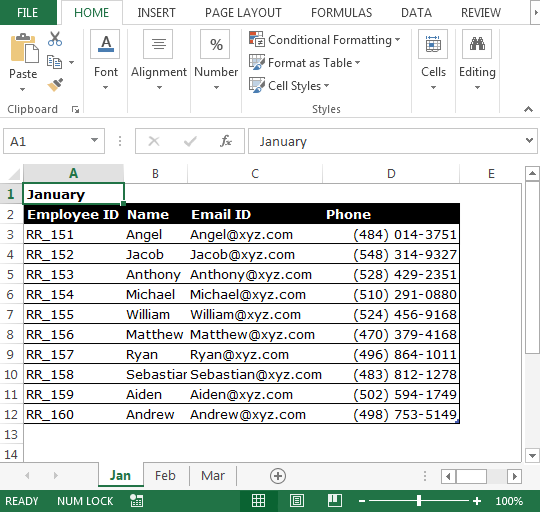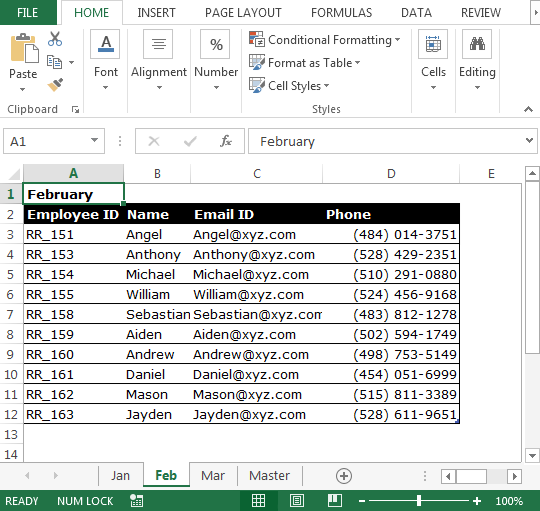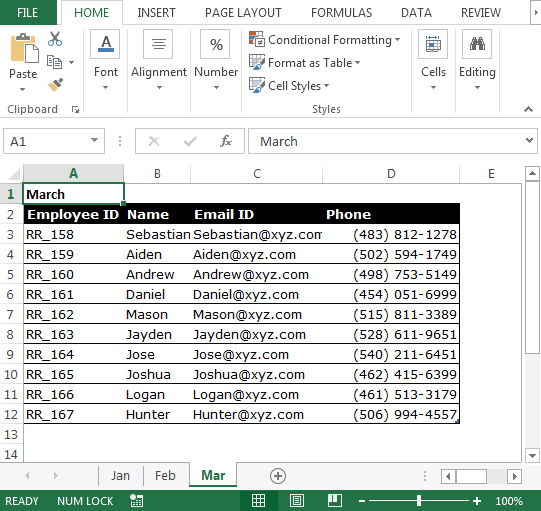To combine data from all the sheets into one sheet, we need to follow the below steps to launch VB editor:

• Click on Developer tab
• From Code group select Visual Basic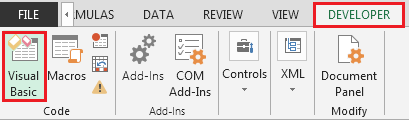• Copy the below code in the standard module

Sub CopyCurrentRegion()
Dim sh As Worksheet
Dim DestSh As Worksheet
Dim Last As Long
If SheetExists("Master") = True Then
MsgBox "The sheet Master already exist"
Exit Sub
End If
Application.ScreenUpdating = False
DestSh.Name = "Master"
For Each sh In ThisWorkbook.Worksheets
If sh.Name <> DestSh.Name Then
If sh.UsedRange.Count > 1 Then
Last = LastRow(DestSh)
sh.Range("A1").CurrentRegion.Copy DestSh.Cells(Last + 1, 1)
End If
End If
Next
Application.ScreenUpdating = True
End Sub

Sub CopyCurrentRegionValues()
Dim sh As Worksheet
Dim DestSh As Worksheet
Dim Last As Long
If SheetExists("Master") = True Then
MsgBox "The sheet Master already exist"
Exit Sub
End If
Application.ScreenUpdating = False
DestSh.Name = "Master"
For Each sh In ThisWorkbook.Worksheets
If sh.Name <> DestSh.Name Then
If sh.UsedRange.Count > 1 Then
Last = LastRow(DestSh)
With sh.Range("A1").CurrentRegion
DestSh.Cells(Last + 1, 1).Resize(.Rows.Count, _
.Columns.Count).Value = .Value
End With
End If
End If
Next
Application.ScreenUpdating = True
End Sub

Function LastRow(sh As Worksheet)
On Error Resume Next
LastRow = sh.Cells.Find(What:="*", _
After:=sh.Range("A1"), _
Lookat:=xlPart, _
LookIn:=xlFormulas, _
SearchOrder:=xlByRows, _
SearchDirection:=xlPrevious, _
MatchCase:=False).Row
On Error GoTo 0
End Function

Function Lastcol(sh As Worksheet)
On Error Resume Next
Lastcol = sh.Cells.Find(What:="*", _
After:=sh.Range("A1"), _
Lookat:=xlPart, _
LookIn:=xlFormulas, _
SearchOrder:=xlByColumns, _
SearchDirection:=xlPrevious, _
MatchCase:=False).Column
On Error GoTo 0
End Function

Function SheetExists(SName As String, _
Optional ByVal WB As Workbook) As Boolean
On Error Resume Next
If WB Is Nothing Then Set WB = ThisWorkbook
SheetExists = CBool(Len(Sheets(SName).Name))
End Function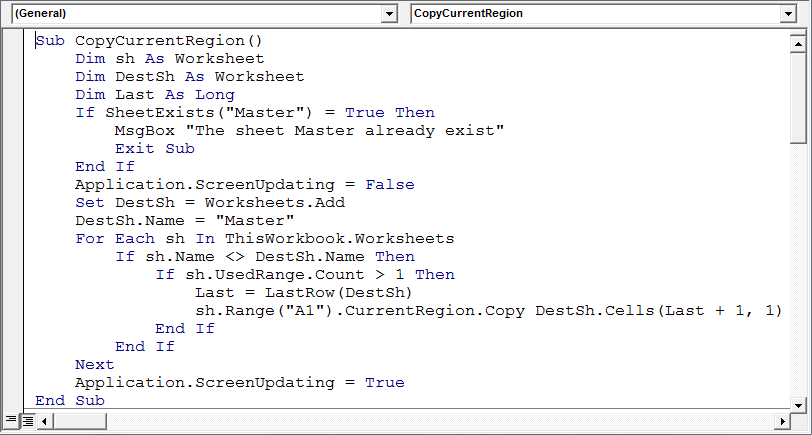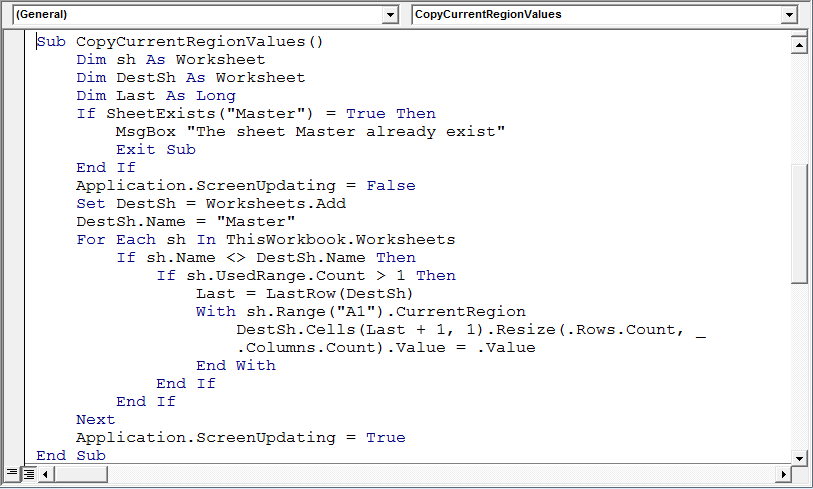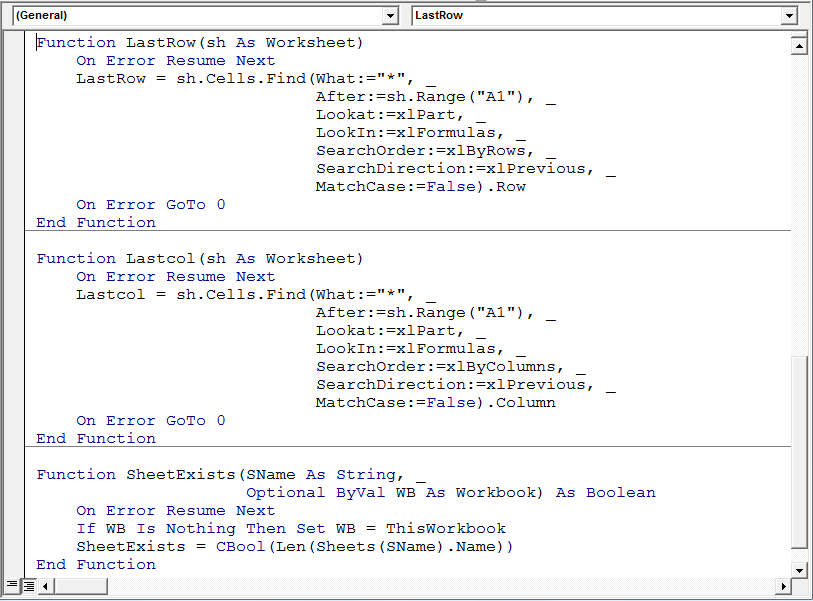CopyCurrentRegion macro will call “SheetExists” function & will check if there is a worksheet name having “Master”; if found then it will do nothing, else it will insert new worksheet in the activeworkbook & rename it to “Master” and then it will copy data from all the sheets.

Following are the snapshots of consolidated data: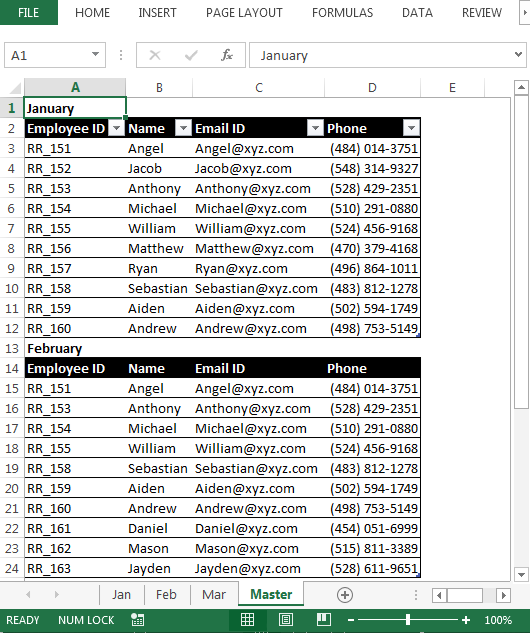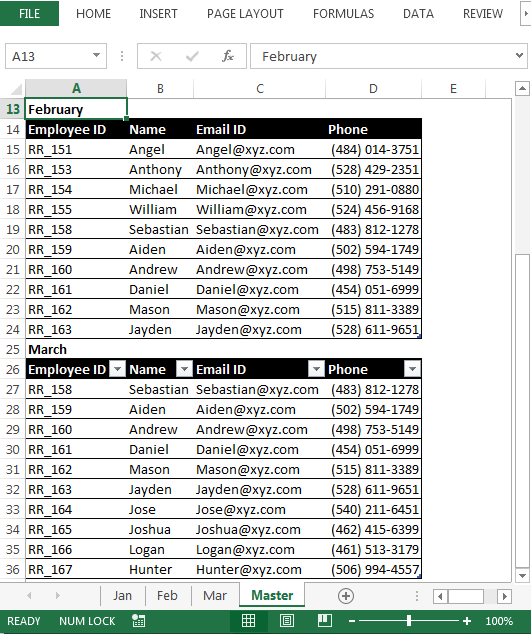Note: The sample workbook contains Master worksheet; it is suggested to delete the Master worksheet & then run the macro to see the VBA code working.

Conclusion: Now we have the code we can use to transfer data from each worksheet into one sheet.